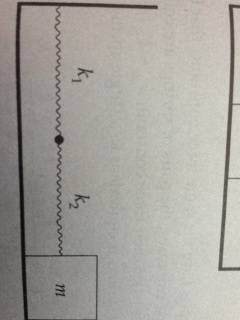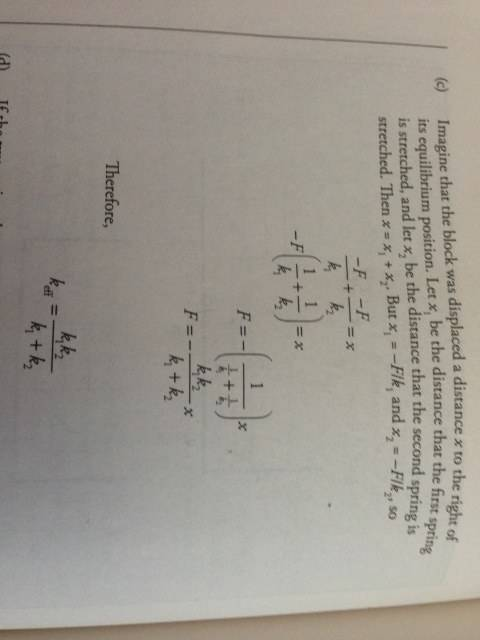# Combining Spring Constants

## Homework Statement

For the following arrangement of two springs, determine the effective spring constant, keff. This is the force constant of a single spring that would produce the same force on the block as the pair of springs shown for this case.(Spring 1 is attached to Spring 2 which is attached to a block of mass m)

Solution Provided by Book:

Imagine that the block was displaced a distance x to the right of its equilibrium position. Let x1 be the distance that the first spring is stretched and let x2 be the distance that the second spring is stretched. Then $x = x_1+ x_2$. But $x_1 = \frac {-F}{k_1}$ and $x_2 = \frac{-F}{k_2}$, so

$\frac{-F}{k_1}+ \frac{-F}{k_2} = x$

$-F(\frac{1}{k_1}+ \frac{1}{k_2}) = x$

$F = \frac{1}{\frac{1}{k_1} + \frac{1}{k_2}}x$

$F = -\frac{k_1k_2}{k_1+k_2}x$

Therefore,

keff = $\frac{k_1k_2}{k_1+k_2}$[/B]
F=-kx

## The Attempt at a Solution

Basically I'm trying to understand how the solution they gave works. I can follow the logic up until the last line of the solution, keff = $\frac{k_1k_2}{k_1+k_2}$.
I understand that each spring exerts the same amount of Force. This is because as x, in F=-kx, increases, k compensates by decreasing.

When I began I thought

Fnew = keff * x

I can solve for x with

$x = x_1 + x_2$

$x_1 = \frac{-F_1}{k_1}$

$x_2 = \frac{-F_2}{k_2}$

$\frac{-F_1}{k_1}+ \frac{-F_2}{k_2} = x$

and if you factor out the F(which you can do because the springs exert the same force) it equals

$-F(\frac{1}{k_1}+ \frac{1}{k_2}) = x$.

Moving it to the other side yields

$F = -\frac{1}{\frac{1}{k_1} + \frac{1}{k_2}}x$

And if you add the fractions they equal

$F = -\frac{k_1k_2}{k_1+k_2}x$

but then they set $F = -\frac{k_1k_2}{k_1+k_2}x$ equal to Fnew = keff * x and come up with the final answer

keff = $\frac{k_1k_2}{k_1+k_2}$

Fnew does not equal F(The force of one spring). How can they set the equations equal to each other?

Last edited:

TSny
Homework Helper
Gold Member
Consider the original system of the two springs connected together. If you pulled on the free end to stretch the system by some distance, how does your force compare to the force that each spring exerts separately? How does your force compare to the force you would exert to stretch the equivalent spring (with keff) the same amount?

I reformatted my post and tried to elaborate a little more because I realized that not even I could understand what I was asking.

My Force = Force of Spring 1 + Force of Spring 2
Force of Spring 1 = Force of Spring 2
My Force = 2F
My Force = Equivalent Spring Force
Equivalent Spring Force = 2F?

But 2F = keff * x cannot be set equal to $F = -\frac{k_1k_2}{k_1+k_2}x$

because the 2nd equation yields the value of the force of only one spring, doesn't it?

Last edited:
TSny
Homework Helper
Gold Member
My Force = Force of Spring 1 + Force of Spring 2

This isn't correct. Suppose you are holding the free end of the two-spring system so the system is stretched at some distance x. Draw a free body diagram for each spring.

Last edited:
I'm having trouble visualizing the forces in the spot between the springs. I know the Force my hand has would be distributed throughout the whole system.

TSny
Homework Helper
Gold Member
In the figure below I have shown the two springs being pulled by your applied force Fap. Below that, I have drawn the two springs separately for the free body diagrams. Can you complete the diagrams by describing what other forces should be included in the free body diagrams?

#### Attachments

Stephen Tashi
My Force = Force of Spring 1 + Force of Spring 2

A spring doesn't exert "a force" (singular). Only when you consider one end of the spring pulling on a free body can you define the force due to the spring. When you use a symbols like "F" without explanation it isn't clear which force you are talking about. If "F" represents the magnitude of several different forces, you should explain that too.

To define what your symbols represent, draw (or describe) some free body diagrams.

TSny
Homework Helper
Gold Member
Good. You are only missing one force that acts on spring #1.

I've scratched my brain but I don't have any idea. Does it have something to do with the opposite force of the applied force?

TSny
Homework Helper
Gold Member
I've scratched my brain but I don't have any idea. Does it have something to do with the opposite force of the applied force?

The left end of the the first spring is attached to the wall. The wall must exert a force on spring #1 as shown below.

Now considering spring #1, what can you say about the magnitudes of the force from the wall and the force from spring #2?

#### Attachments

The left end of the the first spring is attached to the wall. The wall must exert a force on spring #1 as shown below.

Now considering spring #1, what can you say about the magnitudes of the force from the wall and the force from spring #2?

Are they equal?

TSny
Homework Helper
Gold Member
Yes. If an object is at rest and remains at rest, then the net force acting on the object is zero.

What can you say about the two forces acting on the ends of spring #2?

They are also equal?

TSny
Homework Helper
Gold Member
Yes. Think about how the applied force is related to the force given by the formula F2 = k2 x2.

It might help to think about what's going on in the simple case where you stretch a spring a distance x with your left hand holding one end of the spring and your right hand holding the other end. Both hands pull on the spring. How does the force of one of your hands compare to the force given by the formula F = kx?

The amount my hand moves is equal to the x in F=kx.

TSny
Homework Helper
Gold Member
Yes. The important thing is that whenever a spring with spring constant k is stretched by a distance x, each end of the spring is being pulled with a force given by kx.

So, if you look at the free body diagram for spring #2, what is the magnitude of the applied force Fap?
Is it equal to k2 x2 or is it equal to k1 x1 + k2 x2?

It would be just k2x2. The applied force does not exert a force on the first spring. Only the 2nd spring exerts a force on the 1st spring which is equal to the applied force.

TSny
Homework Helper
Gold Member
Right. (And the wall also exerts a force on #1 spring). Good.

So basically does that mean that where ever you are in the system, the force is going to be constant??? Is this why they can refer to the force without any subscript, because they don't have to specify since it's the same everywhere?

TSny
Homework Helper
Gold Member
Yes, I think so. They appear to be thinking of F as the applied force. But that's also equal to the force that spring #1 exerts on #2, the force that spring #2 exerts on #1, and the force that the wall exerts on #1.

Wow. It finally makes sense. Thank you so much!

TSny
Homework Helper
Gold Member
Great. Good luck with your studies.

•Foopyblue